Courses

Trends in Periodic Properties- 1 Notes | Study Inorganic Chemistry - Chemistry

Chemistry: Trends in Periodic Properties- 1 Notes | Study Inorganic Chemistry - Chemistry

The document Trends in Periodic Properties- 1 Notes | Study Inorganic Chemistry - Chemistry is a part of the Chemistry Course Inorganic Chemistry.
All you need of Chemistry at this link: Chemistry

Periodicity in Properties

The periodicity is the repetition of elements with similar properties after certain regular intervals when the elements are arranged in order of increasing atomic number.

• The periodic repetition of properties is due to the recurrence of similar valence shell configurations after regular intervals.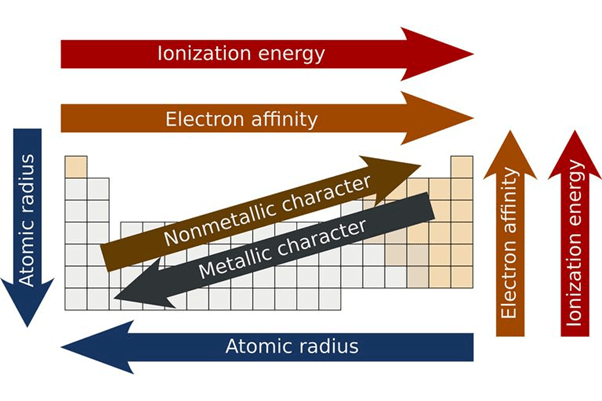Periodicity in the Periodic Table

Atomic Size

Atomic size is the distance between the centre of the nucleus of an atom and its outermost shell.

Factors affecting Atomic Size

Number of Shells

• Atomic Size increases with increase in number of electronic shells. Thus atomic radius is directly proportional to number of electronic shells.
• As n increases, size increases (n = principal of quantum number)

Nuclear Charge

•  As the nuclear charge increases the atomic size decreases due to increase in attractive force. Thus atomic size is inversely proportional to nuclear charge.

Screening Effect

• In an atom having more electrons, particularly more electrons shell, it is observed that the inner orbits decrease the attraction between the electrons in the outer orbit and nucleus.
• Thus they act as screen or shield between electrons of outer orbit and nucleus. This is known as screening effect.
• As the screening effect increases atomic size also increases. Thus atomic radius is directly proportional to screening effect.
• The shield ability of inner electrons decreases in the order of s>p>d>f.

Periodic Trends in Atomic Size

In a Period (Left to Right)

• Atomic size decreases from left to right in a period.
• Looking at the E.C. of elements, the following observations are made:
• The e-s enter in the same shell.
• The number of protons is increasing from left to right with an increase in atomic number.
• The nuclear charge is increasing, so the attractive force of the nucleus on the outermost e is increasing.
• This results in a net contraction of atomic size.

In a Group (up to down)

• Atomic size increases from top to down in a group.
• The electronic configuration of elements of the same group indicates that e-s are added in the new shell in the senior atom, i.e. a new shell is added in the next higher group.
• Although no. of protons are also increasing (i.e. increase in nuclear charge) but the net result is an increase in the size of the atom.
• The net attractive force of the nucleus decreases down the group.

An atomic radius is half the distance between adjacent atoms of the same element in a molecule.

• An atom does not have any sharp boundary, so the atomic radius is determined by the indirect method in the combined state of an atom.
• The atomic radii of atoms of compounds are classified as:
(i) Covalent radii (in covalent molecules)
(ii) Ionic radii (in ionic crystals)
(iii) Van-der-wall radii (in atomic crystals)

Variation in Atomic Radius in a Group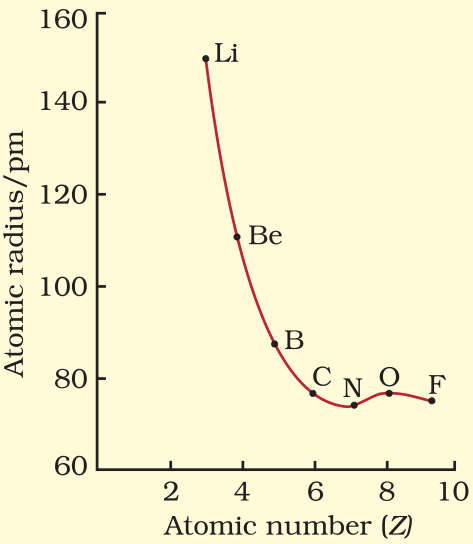Try yourself:Atomic radii ____________ along the periods.

The distance between the nucleus and the outermost shell of an ion is known as ionic radii.

• A cation is smaller than the parent atom and an anion is larger than the parent atom.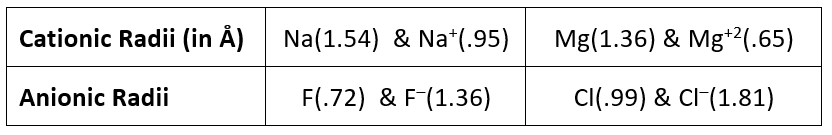• Isoelectronic ions have the same number of electrons.
• Al3+, Mg2+, Na+, Ne, F, O2 and N3 all have 10 electrons.
All have the same configuration: 1s2 2s2 2p6 (which is the noble gas: Neon).
Positive ions that have more protons would be smaller (more protons would pull the same number of electrons).
• For isoelectronic species, size depends upon Z, more Z less size.
• Size:
(i) C+4 < B+3 < Be2+ < Li+
(ii)  Al3+ < Mg2+ < Na+ < Ne < F < O2– < N3–

Ionisation Energy

Amount of energy required to remove the most loosely bounded electron from an isolated gaseous atom.

• Ionisation is endothermic (endoergic) i.e. requires energy hence,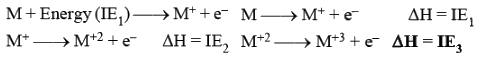• IE3 > IE2 > IE1 always.
• Ionization energy is also called Ionization Potential (l.P.).

Unit of Ionisation Energy

• lonisation energy is expressed in the units of energy, i.e. (eV) per atom; (kJ mol-1);  kcal mol-1.
• 1 eV/atom = 1.602 x 10-16 kJ / atom = 1.602 x 10-16 x 6.02 x 1023 kJ / mol = 96.4 x10kJmol -1

Factors Affecting Ionisation Energy

(i) Size of Atom: Varies inversely

• As the size of the atom increases, the outermost electrons are less tightly held by the nucleus.
• As a result, it is easier to remove the e-.
• The ionisation energy decreases with an increase in atomic size.

(ii) Nuclear Charge: Varies directly

• As nuclear charge increases, it becomes difficult to remove the e-, so ionisation energy increases.

(iii) Screening Effect: Varies inversely

(iv) Nature of orbitals containing e-s: (Penetrating effect of e-s).

(v) Electronic Configuration (E.C.): The atoms having stable E.C. have less tendency to lose electrons. So they have high I.E.

• Half filled E.C.:   N = 1s2, 2s2, 2p
• Full filled E.C. (Noble gas configuration):   Ne = 1s2, 2s2, 2p6
• E.C. with all e-s paired: E.g. Be = 1s2, 2s2;   Zn = 3d104s2;  Cd = 4d10 5s2;  Hg = 5d106s2

General Trend of Ionisation Energy
1. Across the Period: L → R

• Along period I.E. increases (with some exception) [Zeff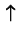­]
• Reason:
(a)  Nuclear Charge­(b)  Atomic Size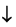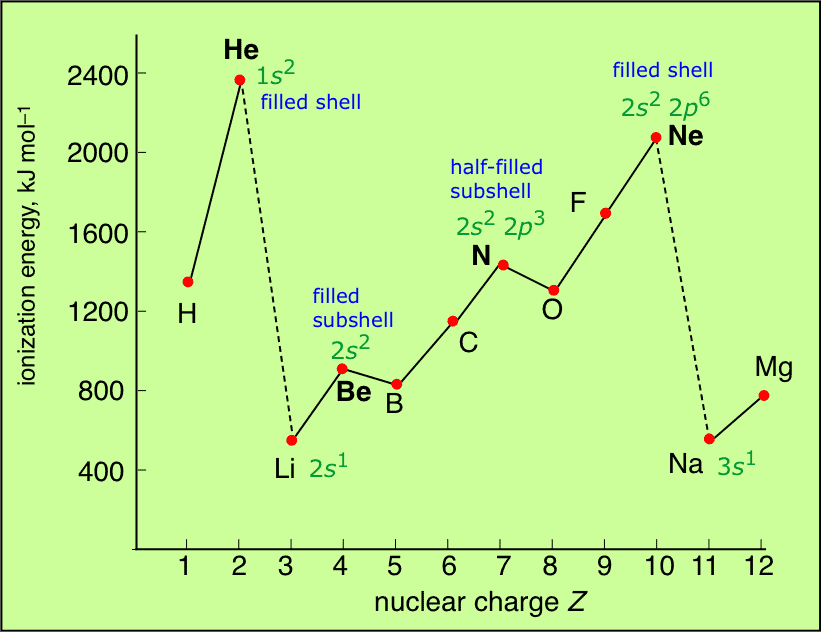2. Along a Group: Top to bottom

• Along a group I.E. decrease [Zeff constant, n­ ]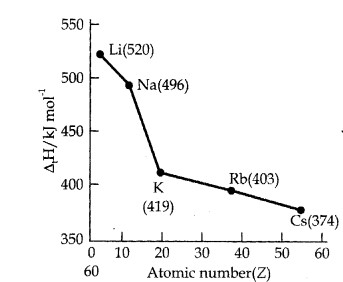• Reason:
(a) Atomic size increases.
(b) Increase in screening effect of electrons of inner shells also increase which nullify the increased Nuclear charge.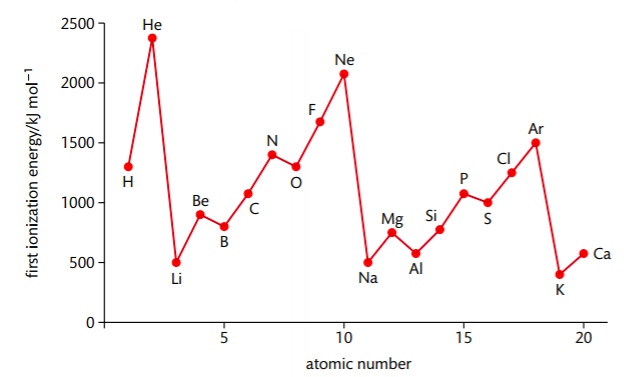First Ionisation Energies of the Elements

Try yourself:What is the trend of ionization energy?

Conclusion

• Maxima and minima occur at the noble gases and alkali metals respectively indicating special stability associated with the closed-shell configuration of noble gases.
• The variation in I.E. along a series of transition metal or inner transition element is much less than that along a period of non-transition elements.
• Half filled E.C. have higher I.E. than the next element along a period (N > O) (P > S).
• Determination of I.E.: I.E. is the difference between the energy of a gaseous atom and that of the system, gaseous ion + free e.
(i) Li < B< Be<C <O < N < F < Ne
(ii) Na < Al < Mg < Si < S < P < Cl < Ar
(iii) K < Ga < Ca < Ge < Se < As < Br < Kr
(iv) Rb < Sr < In < Sn < Sb < Te < I < Xe
(v) Cs < Ba < Tl < Bi < Pb < Po < At < Rn
• I.E. variation in transition metals with dn E.C.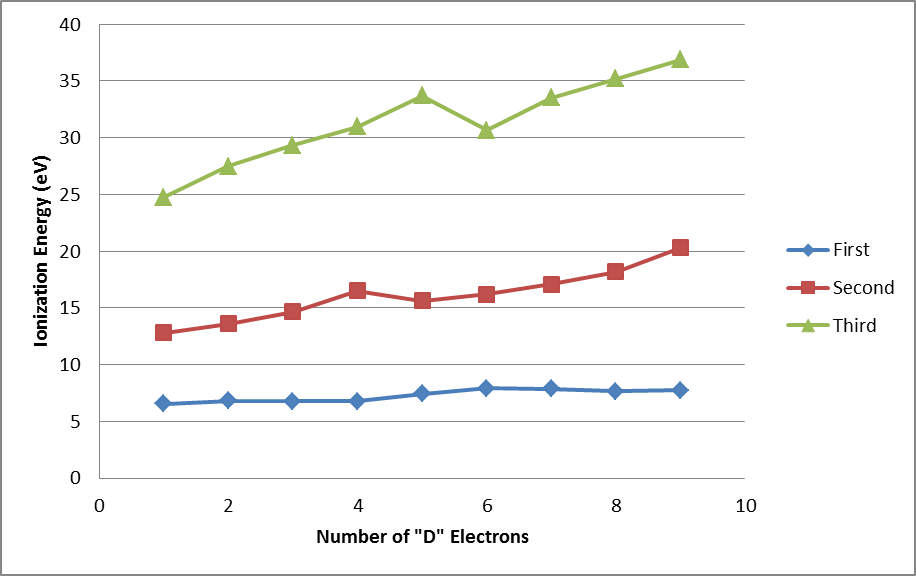• Exceptions
(i) Along a period, half-filled and fully filled have higher I.E.
e.g. Be > B and N > O.
(ii) Along a group, Ga > Al.
• Properties Affected by Ionisation Energy
(i) Metallic character: More ionisation energy means less metallic character (in a period).
(ii) Tending to stay in which state A+1, A+2 or A+3 is stable.

The document Trends in Periodic Properties- 1 Notes | Study Inorganic Chemistry - Chemistry is a part of the Chemistry Course Inorganic Chemistry.
All you need of Chemistry at this link: ChemistryUse Code STAYHOME200 and get INR 200 additional OFF Use Coupon Code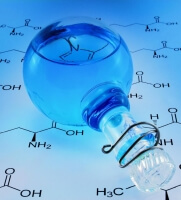Inorganic Chemistry

49 videos|71 docs|16 tests

Track your progress, build streaks, highlight & save important lessons and more!

,

,

,

,

,

,

,

,

,

,

,

,

,

,

,

,

,

,

,

,

,

;